#DAY 22
1
Software Development

## [2020鐵人賽Day22]糊裡糊塗Python就上手-數據化圖之術(上)

### What is Matplotlib?

Matplotlib 是 Python 在 2D 繪圖範疇上使用最廣泛的模組，可以讓開發者快速請簡易的將資料圖形化，並且提供許多樣式的輸出格式

### 實際體驗＆細說觀念

#### Install Matplotlib

``````pip install matplotlib
``````

#### 導入 Matplotlib

``````import matplotlib.pyplot as plt
``````

#### 繪製折線圖 Plot

``````plt.plot(x座標串列值, y座標串列值, [其餘參數值])
``````

``````x = [1, 5, 9, 13, 17]
y = [5, 30, 15, 35, 5]
``````

``````plt.plot(x, y, color='red')
``````

plt.xlabel('x label') # 設定 x 軸標題
plt.ylabel('y label') # 設定 y 軸標題
plt.show()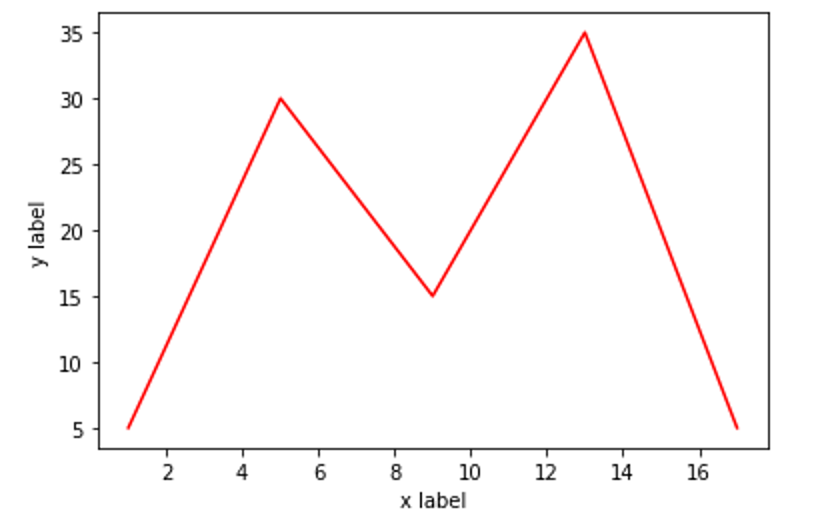#### 其他參數介紹

linewidth 縮寫 lw 設定線條寬度，預設為 1.0 linewidth=3.0
color 設定線條顏色 color="red"
linestyle 縮寫 ls 設定線條樣式，設定值有實線「-」、虛線「--」、虛點線「-.」、點線「:」 linestyle="-."
marker 設定標記樣式，可設定的值有很多，這邊僅取幾個較常用的，「. 點」、「o 圓」、「* 星」、「s 矩形」 marker="*"
markersize 縮寫 ms 標記大小 ms=16
label 設定圖例名稱，需搭配 legend 函式才有效果 label="Test"

#### linestyle 虛線樣式

``````plt.plot(x, y, color='red', linestyle="--")
``````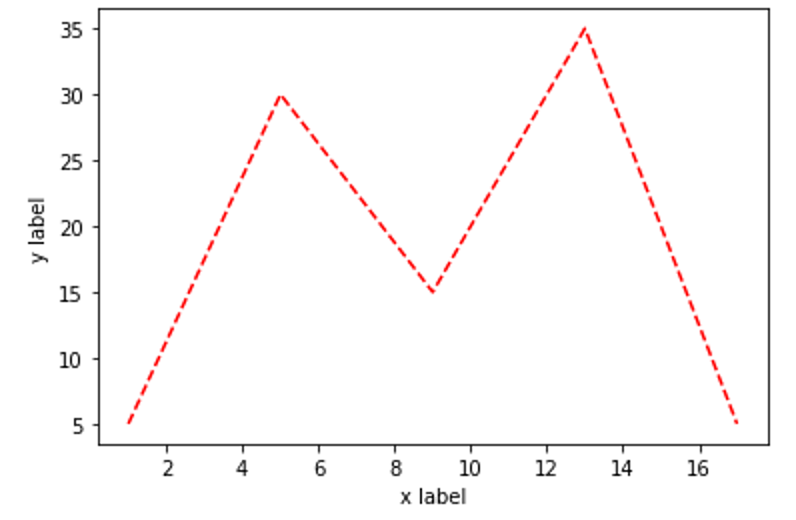#### linestyle 虛點樣式

``````plt.plot(x, y, color='red', linestyle="-.")
``````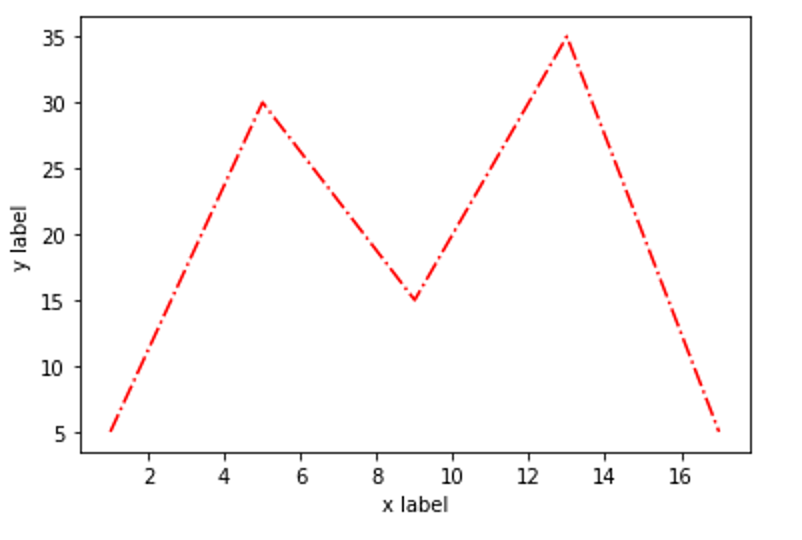#### linestyle 虛點樣式「:」

``````plt.plot(x, y, color='red', linestyle=":")
``````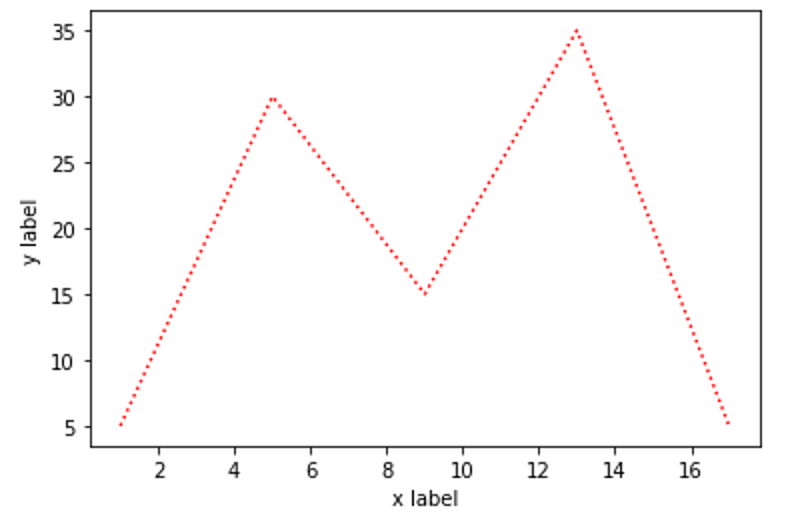#### marker 點「.」標記

``````plt.plot(x, y, color='red', linestyle="-", linewidth="2", markersize="16", marker=".")
``````#### marker 圓「o」標記

``````plt.plot(x, y, color='red', linestyle="-", linewidth="2", markersize="16", marker="o")
``````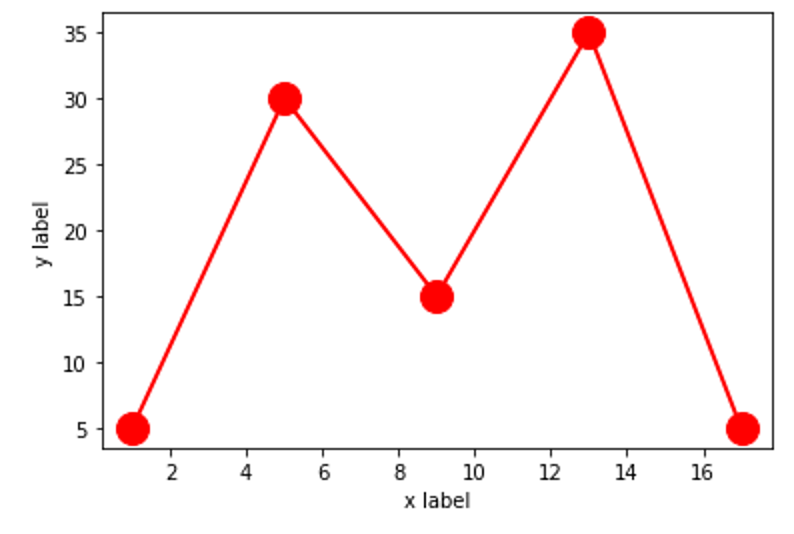#### marker 星「*」標記

``````plt.plot(x, y, color='red', linestyle="-", linewidth="2", markersize="16", marker="*")
``````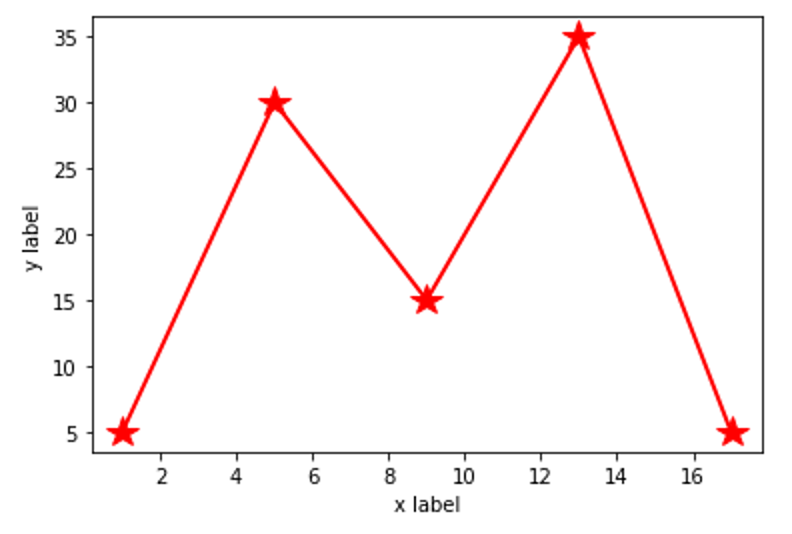#### marker 矩形「s」標記

``````plt.plot(x, y, color='red', linestyle="-", linewidth="2", markersize="16", marker="s")
``````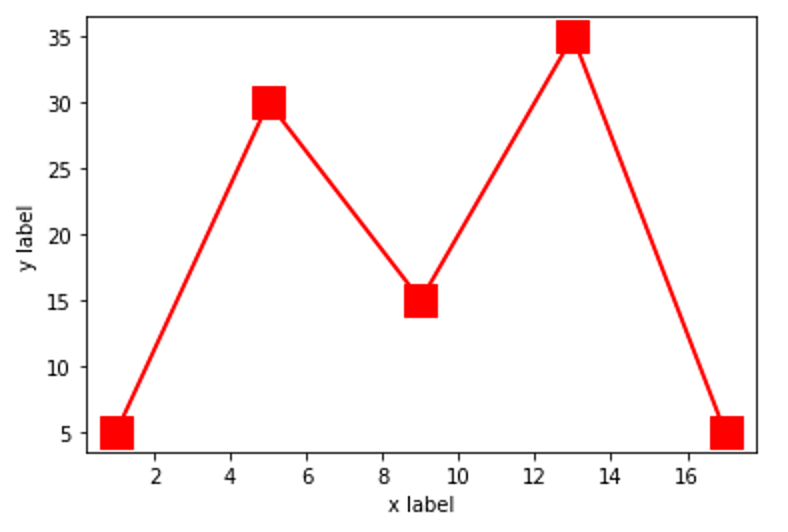#### 設定圖例名稱為「Test」，並設定圖表名稱為「Test title」

``````plt.title('名稱', 字體大小)
``````

``````plt.plot(x, y, color='red', linestyle="-", linewidth="2", markersize="16", marker=".", label="Test")

plt.xlabel('x label', fontsize="10")
plt.ylabel('y label', fontsize="10")
plt.title('Test title', fontsize="18")
``````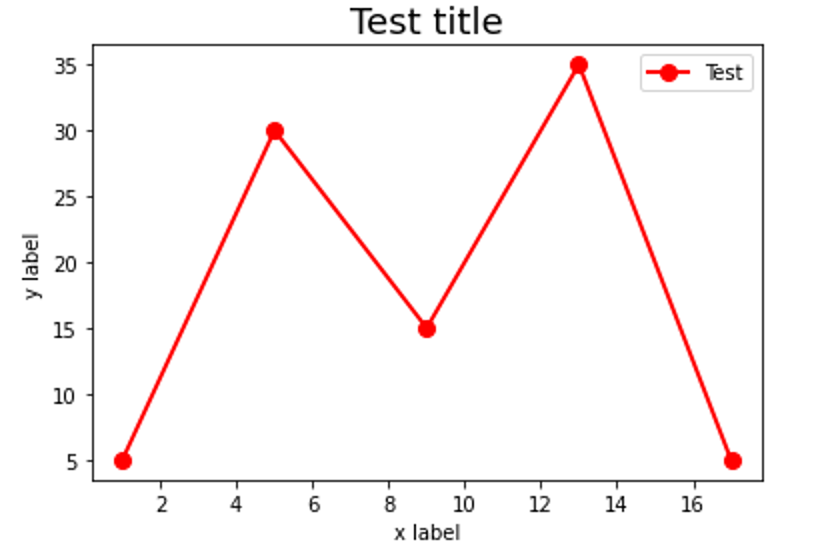#### 其實 x, y 軸都可以設定要顯示的範圍值

``````plt.xlim(起始值, 終止值)
plt.ylim(起始值, 終止值)
``````

``````plt.xlim(0, 30) # 設定 x 軸座標範圍
plt.ylim(0, 50) # 設定 y 軸座標範圍
``````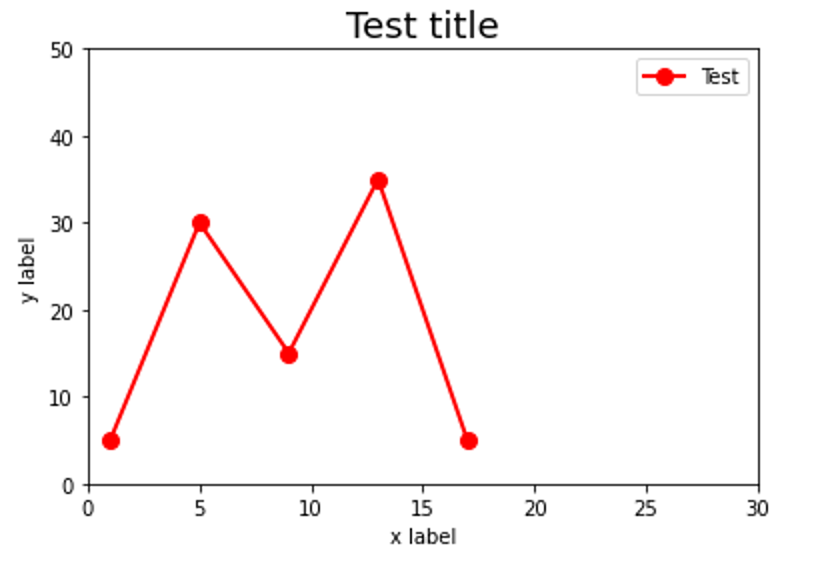### 彙整上述技巧實際演練

``````import matplotlib.pyplot as plt

x1 = [1, 5, 9, 13, 17]
y1 = [5, 30, 15, 35, 5]
# 繪製折線圖，顏色「紅色」，線條樣式「-」，線條寬度「2」，標記大小「16」，標記樣式「.」，圖例名稱「Plot 1」
plt.plot(x1, y1, color='red', linestyle="-", linewidth="2", markersize="16", marker=".", label="Plot 1")

x2 = [3, 8, 12, 16, 20]
y2 = [8, 33, 18, 38, 8]
# 繪製折線圖，顏色「藍色」，線條樣式「-」，線條寬度「2」，標記大小「16」，標記樣式「.」，圖例名稱「Plot 2」
plt.plot(x2, y2, color='blue', linestyle="-", linewidth="2", markersize="16", marker=".", label="Plot 2")

plt.xlim(0, 30) # 設定 x 軸座標範圍
plt.ylim(0, 50) # 設定 y 軸座標範圍

plt.xlabel('x label', fontsize="10") # 設定 x 軸標題內容及大小
plt.ylabel('y label', fontsize="10") # 設定 y 軸標題內容及大小
plt.title('Plot title', fontsize="18") # 設定圖表標題內容及大小

plt.legend()
plt.show()
``````